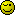# I am saving operators(+,-,*) in mysql table have type varchar i have problem

I am saving operators(+,-,*) in mysql table have type varchar i have problem when in puting the values in formul e.g

\$val1=7;
\$val2=+;
\$val3=5;
\$val4=-;
\$val5=9;

\$ans=\$val1.\$val2.\$val3.\$val4.\$val5;

the output is:

7+5-9

but i want to perform the action and give me the result 3

how to do?

I can’t think of another way to do that besides forming a valid PHP assignment as a string and using eval().

thanks oddz

its not working …giving parse error

what do you want to do with said value?

After you have verified that the input consists of digits, operators and parentheses only, of courseWeird, I tried this but it didn’t work. Either I’m missing something very basic here, or… well, I must be something really basic``````
<?php

\$val0 = '\$res=';
\$val1 = 7;
\$val2 = '+';
\$val3 = 5;
\$val4 = '-';
\$val5 = 9;
\$val6 = ';';

\$sum = \$val0 . \$val1 . \$val2 . \$val3 . \$val4 . \$val5 . \$val6;

echo \$sum . "<br />";
eval('<?php ' . \$sum . ' ?>');

var_dump(isset(\$res));

?>

``````

Output:

``````

\$res=7+5-9;

boolean false

``````

p.s. I have, of course, checked that the input consists of digits, operators and parentheses onlyYou don’t put <?php and ?> in the eval, just the code you want to evaluate.

``````
<?php
\$val0 = '\$res=';
\$val1 = 7;
\$val2 = '+';
\$val3 = 5;
\$val4 = '-';
\$val5 = 9;
\$val6 = ';';

\$sum = \$val0 . \$val1 . \$val2 . \$val3 . \$val4 . \$val5 . \$val6;

echo \$sum . "<br />";
eval(\$sum);

var_dump(isset(\$res));
var_dump(\$res);

/*
\$res=7+5-9;
boolean true
int 3
*/

``````if the values are coming from user input you might have to do more checks, i.e.

``````\$val0 = '\$res=';
\$val1 = '/'; // user supplied
\$val2 = '/'; // user supplied
\$val3 = ';';
``````

eval() now throws a parse error

Of course, that just goes to show how often I use eval().